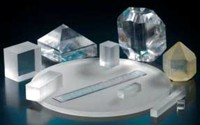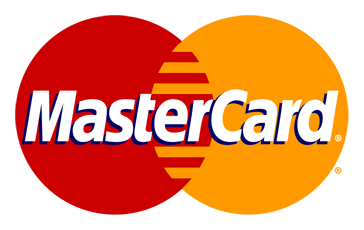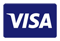Search for:

# Potassium Dihydrogen Phosphate / Potassium Dideuterium Phosphate (KDP, DKDP or KD*P)Potassium Dihydrogen Phosphate (KDP) and Potassium Dideuterium Phosphate (DKDP) are nonlinear optical materials (NLO) know for their large NLO coefficients, wide transparency range and high optical damage threshold. These materials are widely used as frequency multipliers for generation of the second, third and fuorth harmonics for Nd:YAG, Nd:YLF lasers. Both crystals are also applied in electro-optics: as Q-switches for Nd:YAG, Nd:YLF, Ti:sapphire and Alexandrite lasers and Pockels cells. Owing to a posibility of growing large crystals, high laser frequency multipliers. Q-switches and Pockels cells, laser damage threshold of witch is over 40 J/cm2 (at lambda=1,06 µm, tau=3 ns). This allows to use them in the devices for laser fusion. KDP and DKDP crystals possess elctro-optical, nonlinear and piesoelectric properties, high laser damage threshold and are used as the base for the devices and systems of laser engineering: frequency multipliers, modulators, gates, deflectors.
 Basic Properties45 KDP DKDP Chemical Fomula KH2PO4 KD2PO4 Class of symmetry 42m 42m Crystal structure tetragonal tretagonal Transparency Range, mm 0,2 – 1,5 0,2 – 1,6 Wafelength range, nm 200-1500 2 Electro – optical Coefficients, pm/V-1. r41 = 8.8r63 = 10.3 r41 = 8.83 = 25 Nonlinear Coefficients d36 = 0.44 pm/V d36 = 0.40 pm/V Refractive index (at 1064 nm) n0 = 1.4938 nc = 1.4599 no = 1.4948nc = 1.4554 Temperature Synchronism Width, oC*cm 11,5. 7,4. Spectral Synchronism Width, A *cm 106 Angle Synchronism Width, mrad*cm 0,84 0,94 Longitudinal half-wave voltage Vn = 7.65 KV (l = 546 nm) Vn = 2.98 KV (l= 546 nm) Absorpion Coefficient, cm-1 0.07 0.006 Optical Damage Threshold(l=1,064 mm, t=10ns) >5 GW / cm² >3 GW / cm² ohs Hardness 2,5 2,5 Extinction ratio 30 dB Sellmeier equations of DKDP n02 = 1.9575544 + 0.2901391 l2 / (l2 – 0.0281399) – 0.02824391 l2 + 0.004977826 l2 nc2 = 1.5005779 + 0.6276034 l2 / (l2 – 0.0131558) – 0.01054063 l2 + 0.002243821 l4 Sellmeier equations of KDP n02 = 2.259276 + 0.01008956 / (l2 – 0.012942625) + 13.00522 l2 / (l2 – 400) nc2 = 2.132668 + 0.008637494 / (l2 – 0.012281043) + 3.2279924 l2 / (l2 – 400)
 Refractive Index Sellmeier Equation n2 = A + B / (l2 – C) + Dl2 / (l2 – E) (l in mm) Sellmeier Coeficients KDP KD*P ADP CDA CD*A A no 2.2576 2.2409 2.3041 0.4204 2.4082 ne 2.1295 2.1260 2.1643 2.3503 2.3458 B no 0.0101 0.0097 0.0111 0.0163 0.0156 ne 0.0097 0.0086 0.0097 0.0156 0.0151 C no 0.0142 0.0156 0.0133 0.0180 0.0191 ne 0.0014 0.0120 0.0129 0.0168 0.0168 D no 1.7623 2.2470 15.1086 1.4033 2.2122 ne 0.7580 0.7844 5.8057 0.6853 0.6518 E no 57.8984 126.9205 400.0000 57 8239 126.8709 ne 127.0535 123.4032 400.0000 127.2700 127.3309 l = 1064 no 1.4942 1.4931 1.5071 1.5515 1.5499 TypicalValues nm ne 1.4603 1.4583 1.4685 1.5356 1.5341 l = 532 no 1.5129 1.5074 1.5280 1.5732 1.5692 TypicalValues nm ne 1.4709 1.4683 1.4819 1.5516 1.5496 l = 355 no 1.5317 1.5257 1.5487 1.6026 1.5974 nm ne 1.4863 1.4833 1.4994 1.5788 1.5759

 We accept credit-cardsSurfaceNet GmbH | Oskar-Schindler-Ring 7 | 48432 Rheine | Germany Phone: +49 (0) 5971 4010179 E-Mail: sales@surfacenet.de © SurfaceNet GmbH